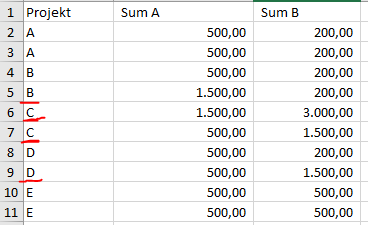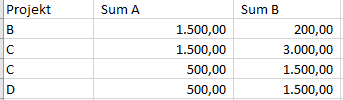# Multiple Row Filter

Hello,

can someone help me with a simple filter:

Assume the following Data:I would like to filter the colums if the value is in Sum A and/or in Sum B bigger than 1000

So the result should look like:Which node and which settings I should use best?

Thank you for help
BR

1 Like

Hallo @Markus3003

A possible solution is as follows using the -Rule-Based Row Filter- node:

``````\$Sum A\$ > 1000 OR \$Sum B\$ > 1000 => TRUE
TRUE => FALSE
``````

Given that every condition is evaluated and executed in the order you write them, another simple way of coding this is as follows:

``````\$Sum A\$ > 1000 => TRUE
\$Sum B\$ > 1000 => TRUE
TRUE => FALSE
``````

Both are equivalent. Be aware that the last condition “TRUE => FALSE” is not needed if you use a -Rule-Based Row Filter- node but would be necessary if you use a -Rule-Based Row Splitter- instead to get the filtered off rows available at the 2nd node output.

Hope it helps.

Best
Ael

4 Likes

Rule-based Row Filter does the trick via `\$Sum A\$ > 1000 OR \$Sum B\$ > 1000 => TRUE`

3 Likes

This topic was automatically closed 7 days after the last reply. New replies are no longer allowed.